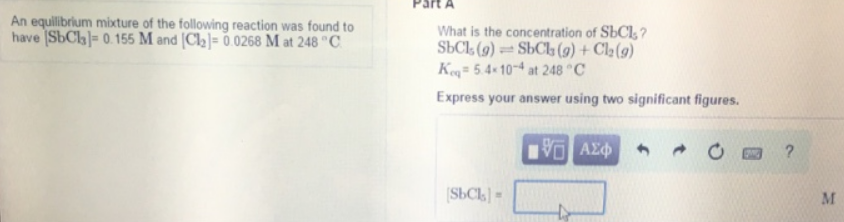# Problem: An equilibrium mixture of the following reaction was found to have [SbCl3] = 0.155 M and [Cl2] = 0.0268 M 248°CWhat is the concentration of SbCl5?SbCl5(g) ⇌ SbCl3(g) + Cl2(g)Keq = 5.4x 10-4 at 248°CExpress your answer using two significant figures.

###### FREE Expert Solution
82% (413 ratings)###### Problem Details

An equilibrium mixture of the following reaction was found to have [SbCl3] = 0.155 M and [Cl2] = 0.0268 M 248°C

What is the concentration of SbCl5?

SbCl5(g) ⇌ SbCl3(g) + Cl2(g)
Keq = 5.4x 10-4 at 248°C